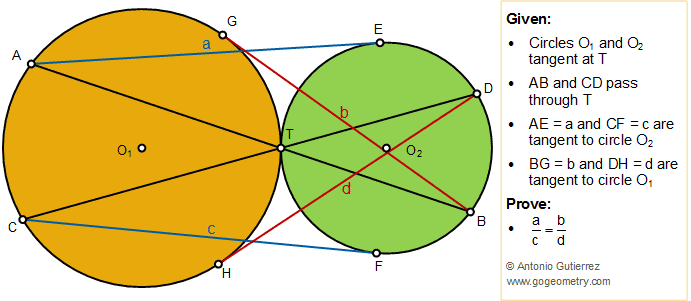## Sunday, May 5, 2019

### Geometry Problem 1432: Tangent Circles, Secant, Tangent Lines, Proportionality, Similarity

Geometry Problem. Post your solution in the comment box below.
Level: Mathematics Education, High School, Honors Geometry, College.

Details: Click on the figure below.1.https://photos.app.goo.gl/uWKn5PxzEsWQnLBy9

Let R=TO1 and r=TO2
Let 2 α= angle AO1T= angle TO2B
In triangle AO1O2, using cosine formula we have
AO2^2= (R+r)^2+R^2-2R(R+r)cos(2 α)
And AE^2=a ^2=AO2^2-r^2
AE^2=2R(R+r)(1-cos2 α)
Replace 1-cos(2 α)=2 sin(α)^2 in above we get
AE^2= 4R(R+r)sin(α)^2 => a= 2.sqrt(R(R+r)).sin(α)
In the same way we have BG= b= 2.sqrt(r(R+r)).sin(α)
So a/b= sqrt(R/r)
And ratio of tangent from A to circle O2 and B to circle O1 is not depend on angle α
So c/d= sqrt(R/r) and a/b=c/d or a/c=b/d

2.connect AC, BD;
Through T, make common tangent line.
It is easy to see triangle ACT similar to triangle BDT;
so AT/CT = BT/DT ;
ALSO a^2 = AT*AB; c^2 = CT*CD;
b^2 = BT*AB; d^2 = DT*CD;
SO a^2/c^2 = b^2/d^2
so a/c= b/d

3.By drawing the common tangent at T we can easily deduce that AC//BD

C^2/d^2 = CT.CD/DT.CD = CT/DT = AT/BT (because AC//BD) = AT.AB/BT.AB = a^2/b^2

From which a/c = b/d

Sumith Peiris
Moratuwa
Sri Lanka